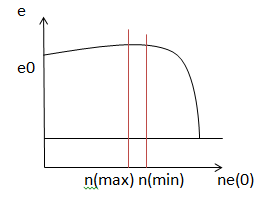# Dielectric constant

A dielectric material is an electrical insulator that can be polarized by an applied electric field. When a dielectric is placed in an electric field, electric charges do not flow through the material as they do in a conductor, but only slightly shift from their average equilibrium positions causing dielectric polarization.It is also known as Relative permittivity. If two charges q 1 and q 2 are separated from each other by a small distance r. Then by using the coulombs law of forces the equation formed will be

K = Er = Em / E0

where K is the Dielectric constant and Er is the relative permilitivity , em is the permimlity of the material , E0 is the permitivity of vaccume.

If you are still not clear about the definition of the Dielectric Constant, just hold it on there and just try to understand how it affects physical characteristics that you are familiar with. As you see more and more of those examples, you would get stronger motivation to understand the definition and get back to what is described above. Then, the definition parts would come to you with more intimate face.

If you put a dielectric material with larger dielectric constant between the two plates when you make a capacitor, you would get a capacitor with larger capacitance value.

#### Dielectric polarization

If a material contains polar molecules, they will generally be in random orientations when no electric field is applied. An applied electric field will polarize the material by orienting the dipole moments of polar molecules.This decreases the effective electric field between the plates and will increase the capacitance of the parallel plate structure. The dielectric must be a good electric insulator so as to minimize any DC leakage current through a capacitor.

This can be reduced to a simple dipole using the superposition principle. A dipole is characterized by its dipole moment, a vector quantity shown in the figure as the blue arrow labeled M. It is the relationship between the electric field and the dipole moment that gives rise to the behavior of the dielectric.